# What is the sum of 2 2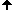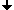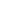### The sum of the first N square numbers

We consider the sum of the first N square numbers, i.e. 1 + 4 + 9 + ... + N2.
 total Factorization 3 * total 2 * 3 * total 1 1 1 1 1 1 1 3 1 2 3 1+4 5 1 1 5 1 3 5 2 3 5 1+4+9 14 1 2 7 3 2 7 3 4 7 1+4+9+16 30 2 5 3 2 5 9 etc 1+4+9+16+25 55 5 1 11 5 3 11 1+4+9+16+25+36 91 1 7 13 3 7 13 1+4+9+16+25+36+49 140 7 4 5 7 4 15 1+4+9+16+25+36+49+64 204 4 3 17 4 9 17 1+4+9+16+25+36+49+64+81 285 3 5 19 9 5 19 1+4+9+16+25+36+49+64+81+100 385 5 11 7 5 11 21 1+4+9+16+25+36+49+64+81+100+121 506 11 2 23 11 6 23 1+4+9+16+25+36+49+64+81+100+121+144 650 2 13 25 6 13 25 1+4+9+16+25+36+49+64+81+100+121+144+169 819 13 7 9 13 7 27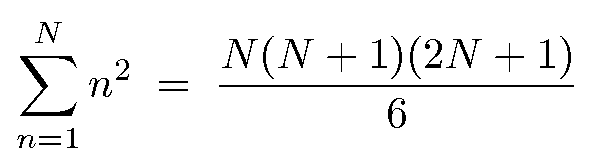This formula is already at Brahmagupta (598-670),
in his book Brahmasphutasiddhanta (628),
but without proof.

Proofs:

• Algebraic: With full induction
• Geometric proof (by Giorgio Goldoni):

Build 6 pyramids of the following shape (here for N = 4):They can be put together to form a cuboid with the edge lengths N, N + 1, 2N + 1.

Here is the assembly of three such pyramids: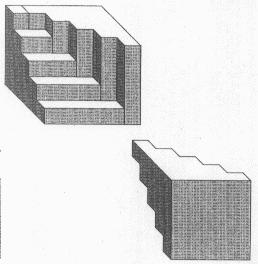A cuboid "with an outside staircase" is obtained.
Obviously, two such cuboids with their external stairs together form a compact cuboid!

For capital N, these pyramids resemble those pyramids known from dividing the cube into thirds by congruent pyramids: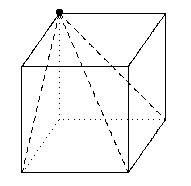In Chinese these are called pyramids Yang-ma, they play an important role, for example, in calculating the volume of truncated pyramids (Liu Hui, 3rd century CE, commentary on the 9 chapters).

The above pyramids, which we used in proving the formula for the sum of the first N square numbers, generalize the geometric proof for the sum of the first N numbers.

Here the case N = 5: